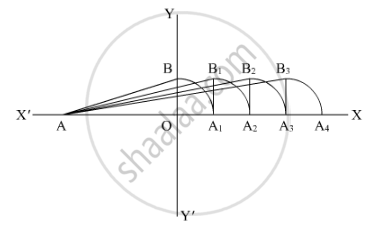Share

# Represent Sqrt6, Sqrt7, Sqrt8 on the Number Line. - CBSE Class 9 - Mathematics

ConceptRepresenting Real Numbers on the Number Line

#### Question

Represent sqrt6, sqrt7, sqrt8 on the number line.

#### Solution

We are asked to represent sqrt6, sqrt7 and sqrt8on the number line

We will follow certain algorithm to represent these numbers on real line

We will consider point A as reference point to measure the distance(1) First of all draw a line AX and YY’ perpendicular to AX

(2) Consider AO = 2 unit and OB = 1 unit, so

AB=sqrt(2^2+1^2)=sqrt5

(3) Take A as center and AB as radius, draw an arc which cuts line AX at A1

(4) Draw a perpendicular line A1B1 to AX such that A1B1 = 1 unit and

(5) Take A as center and AB1 as radius and draw an arc which cuts the line AX at A2.

Here

AB1 = AA2

=sqrt("AA"_1^2+A_1B_1^2)

=sqrt((sqrt5)^2+1)

=sqrt6 unit

So AA2 = sqrt6 unit

So A2 is the representation for sqrt6

(1) Draw line A2B2 perpendicular to AX

(2) Take A center and AB2 as radius and draw an arc which cuts the horizontal line at A3 such that

AB2 = AA3

=sqrt("AA"_2^2+A_2B_2^2)

=sqrt((sqrt6)^2+1)

=sqrt7 unit

So point A3 is the representation of sqrt7

(3) Again draw the perpendicular line A3B3 to AX

(4) Take A as center and AB3 as radius and draw an arc which cuts the horizontal line at A4

Here;

AB3 = AA4

=sqrt("AA"_3^2+A_3B_3^2)

=sqrt((sqrt7)^2+1^2)

=sqrt8 unit

A4 is basically the representation of sqrt8

Is there an error in this question or solution?

#### Video TutorialsVIEW ALL 

Solution Represent Sqrt6, Sqrt7, Sqrt8 on the Number Line. Concept: Representing Real Numbers on the Number Line.
S